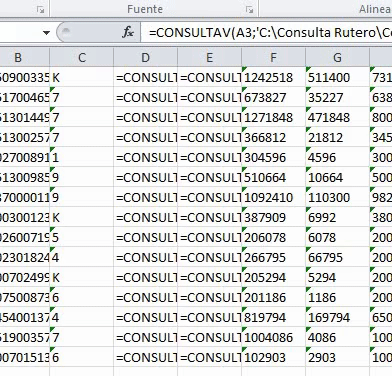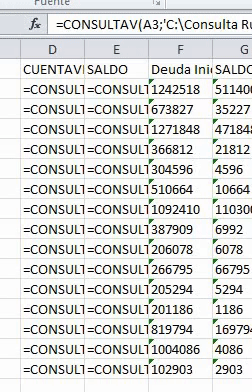# Insert excel formulas from Datatable into Excel as formulas and not as strings

Continuing the discussion from Fastest way to write an excel formula into an entire column:

Hi all

I’m hoping someone could help me to resolve the issue presented in the link above.

The problem I’m having is when I try to write into an excel file a formula from a Datatable, it does not write it as a formula but as a string, thus missing all the point about optimizing.

I tried the hotkey method and doesn’t do what we need, in the excel formulas we are implementing we are calling other workbooks (e.g. =VLOOKUP(A3;‘C:\somerute[Another workbook.xlsx]sheet1’!\$A\$1:\$I\$4097;9;0)). For some reason it only shows the value of the previos cell in the same workbook and not the one that we are looking for. I made a gif to make it more visual:With the Datatable method, we tried both “Write Range” activities (Excel App Scope and Workbook) and with the Excel one it throws the error “Range does not exist”, with the Workbook one it paste the formula as a string (based on this).

For the “range does not exist error” I tried this and it does not work at all.

Please we need to solve this issue, your help is much appreciated.

Regards

UPDATE
Hotkey `Ctrl + D` is for english excel versions and not spanish, the spanish version of the hotkey is `Ctrl + J` (here is more info about spanish excel hotkeys).

Even so, it fills the rows with the formula but still as a string and not as formula:Having the same problem here, I want to paste a formula from a variable into an excel file with a Write Cell, but it writes it as text, so as a string…

Did you find a solution yet by any chance?

Read the cell formula value using ‘Read Cell Formula’ activity say varFormula
Then add one Assign activity and create one more variable ‘concatForumula’ and follow the below
concatForumula = “=”+varFormula

Add ‘Write Cell’ activity, and use the concatFormula variable in the required cell.
Let me know if you still any issues?

3 Likes